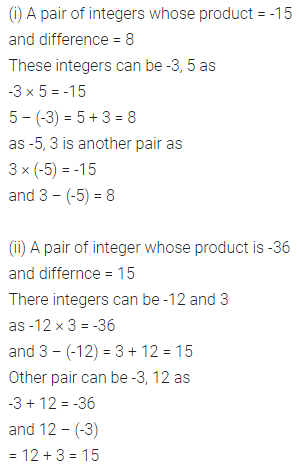# ML Aggarwal Class 7 Solutions for ICSE Maths Chapter 1 Integers Ex 1.3

## ML Aggarwal Class 7 Solutions for ICSE Maths Chapter 1 Integers Ex 1.3

Question 1.
Find the following products:
(i) 7 × (-35)
(ii) (-13) × (-15)
(iii) (-12) × (-11) × (-10)
(iv) (-13) × 0 × (-24)
(v) (-1) × (-2) × (-3) × 4
(vi) (-3) × (-6) × (-2) × (-1)
Solution: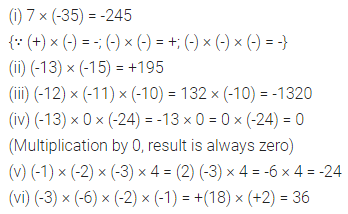Question 2.
Verify the following:
(i) 37 × [6 + (-3)] = 37 × 6 + 37 × (-3)
(ii) (-21) × [(-6) + (-4)] = (-21) × (-6) + (-21) × (-4)
Solution: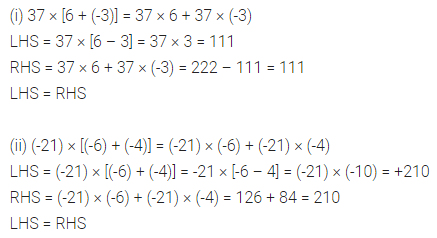Question 3.
Using suitable properties, evaluate the following:
(i) 8 × 53 × (-125)
(ii) (-8) × (-2) × 3 × (-5)
(iii) (-6) × 2 × (-8) × 5
(iv) 15 × (-25) × (-4) × (-10)
(v) 26 × (-48) + (-48) × (-36)
(vi) 724 × (-56) + (-724) × 44
(vii) (-47) × 102
(viii)(-39) × (-97)
Solution: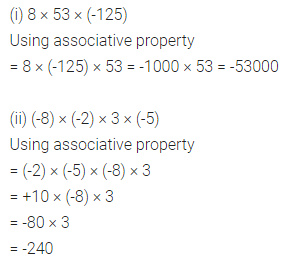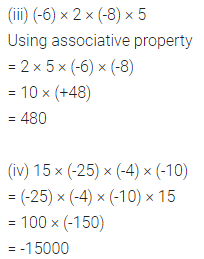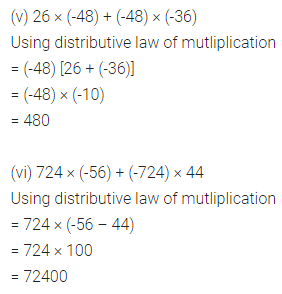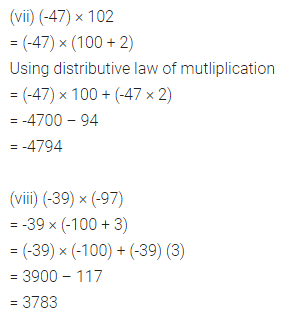Question 4.
Fill in the blanks to make the following true statements:
(i) (-4) × …… = 44
(ii) 7 × …… = -42
(iii) …… × (-13) = 143
(iv) (-5) × …… = 0
Solution:Question 5.
A certain freezing process requires that room temperature be lowered from 32°C at the rate of 5°C every hour. What will be the room temperature 8 hours after the freezing process begins?
Solution: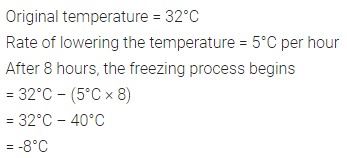Question 6.
In a class test containing 10 questions, 5 marks are awarded for every correct answer and 2 marks are deducted for every incorrect answer and 0 for questions not attempted.
(i) Rohit gets four correct and six incorrect answers. What is his score?
(ii) Seema gets 5 correct and 5 incorrect answers. What is her score?
(iii) Ritu attempted 7 questions and gets only 2 correct answers. What is her score?
Solution:Question 7.
(i) Find a pair of integers whose product is -15 and whose difference is 8.
(ii) Find a pair of integers whose product is -36 and whose difference is 15.
Solution: